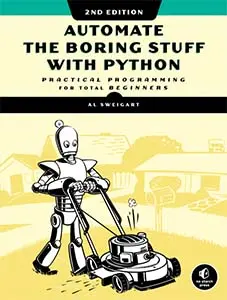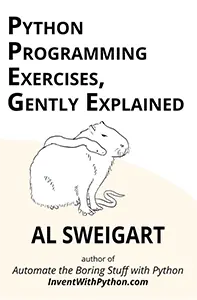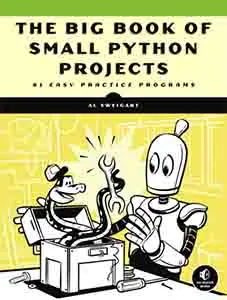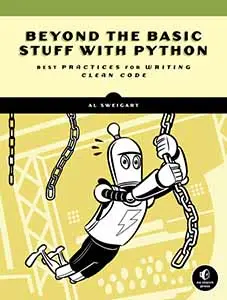# The Invent with Python Blog

Writings from the author of Automate the Boring Stuff.

Mon 09 July 2012

# 16 Common Python Runtime Errors That Beginners Find

Posted by Al Sweigart in python

Figuring out what Python's error messages mean can be kind of tricky when you are first learning the language. Here's a list of common errors that result in runtime error messages which will crash your program.

1) Forgetting to put a : at the end of an `if`, `elif`, `else`, `for`, `while`, `class`, or `def` statement. (Causes “`SyntaxError: invalid syntax`”)

This error happens with code like this:

```if spam == 42

print('Hello!')```

2) Using `=` instead of `==`. (Causes “`SyntaxError: invalid syntax`”)

The `=` is the assignment operator while `==` is the "is equal to" comparison operator. This error happens with code like this:

```if spam = 42:

print('Hello!')```

3) Using the wrong amount of indentation. (Causes “`IndentationError: unexpected indent`” and “`IndentationError: unindent does not match any outer indentation level`” and “`IndentationError: expected an indented block`”)

Remember that the indentation only increases after a statement ending with a `:` colon, and afterwards must return to the previous indentation. This error happens with code like this:

```print('Hello!')

print('Howdy!')```

...and this:

```if spam == 42:

print('Hello!')

print('Howdy!')```

...and this:

```if spam == 42:

print('Hello!')```

4) Forgetting the `len()` call in a `for` loop statement. (Causes “`TypeError: 'list' object cannot be interpreted as an integer`”)

Commonly you want to iterate over the indexes of items in a list or string, which requires calling the `range()` function. Just remember to pass the return value of `len(someList)`, instead of passing just `someList`.

This error happens with code like this:

```spam = ['cat', 'dog', 'mouse']

for i in range(spam):

print(spam[i])```

(Update: As a few have pointed out, what you might need is just `for i in spam:` rather than the above code. But the above is for the very legitimate case where you need the index in the body of the loop, rather than just the value itself.)

5) Trying to modify a string value. (Causes “`TypeError: 'str' object does not support item assignment`”)

Strings are an immutable data type. This error happens with code like this:

```spam = 'I have a pet cat.'

spam = 'r'

print(spam)```

What you probably want is this:

```spam = 'I have a pet cat.'

spam = spam[:13] + 'r' + spam[14:]

print(spam)```

6) Trying to concatenate a non-string value to a string value. (Causes “`TypeError: Can't convert 'int' object to str implicitly`”)

This error happens with code like this:

```numEggs = 12

print('I have ' + numEggs + ' eggs.')```

What you want to do is this:

```numEggs = 12

print('I have ' + str(numEggs) + ' eggs.')```

...or this:

```numEggs = 12

print('I have %s eggs.' % (numEggs))```

7) Forgetting a quote to begin or end a string value. (Causes “`SyntaxError: EOL while scanning string literal`”)

This error happens with code like this:

`print(Hello!')`

...or this:

`print('Hello!)`

...or this:

```myName = 'Al'

print('My name is ' + myName + . How are you?')```

8) A typo for a variable or function name. (Causes “`NameError: name 'fooba' is not defined`”)

This error happens with code like this:

```foobar = 'Al'

print('My name is ' + fooba)```

...or this:

`spam = ruond(4.2)`

...or this:

`spam = Round(4.2)`

9) A typo for a method name. (Causes “`AttributeError: 'str' object has no attribute 'lowerr'`”)

This error happens with code like this:

```spam = 'THIS IS IN LOWERCASE.'

spam = spam.lowerr()```

10) Going past the last index of a list. (Causes “`IndexError: list index out of range`”)

This error happens with code like this:

```spam = ['cat', 'dog', 'mouse']

print(spam)```

11) Using a non-existent dictionary key. (Causes “`KeyError: 'spam'`”)

This error happens with code like this:

```spam = {'cat': 'Zophie', 'dog': 'Basil', 'mouse': 'Whiskers'}

print('The name of my pet zebra is ' + spam['zebra'])```

12) Trying to use a Python keyword for a variable name. (Causes “`SyntaxError: invalid syntax`”)

The Python keywords (also called reserved words) cannot be used for variable names. This happens with code like:

`class = 'algebra'`

The Python 3 keywords are: `and`, `as`, `assert`, `break`, `class`, `continue`, `def`, `del`, `elif`, `else`, `except`, `False`, `finally`, `for`, `from`, `global`, `if`, `import`, `in`, `is`, `lambda`, `None`, `nonlocal`, `not`, `or`, `pass`, `raise`, `return`, `True`, `try`, `while`, `with`, `yield`

13) Using an augmented assignment operator on a new variable. (Causes “`NameError: name 'foobar' is not defined`”)

Do not assume that variables start off with a value such as `0` or the blank string. A statement with an augmented operator like `spam += 1` is equivalent to `spam = spam + 1`. This means that there must be a value in `spam` to begin with.

This error happens with code like this:

```spam = 0

spam += 42

eggs += 42```

14) Using a local variable (with the same name as a global variable) in a function before assigning the local variable. (Causes “`UnboundLocalError: local variable 'foobar' referenced before assignment`”)

Using a local variable in a function that has the same name as a global variable is tricky. The rule is: if a variable in a function is ever assigned something, it is always a local variable when used inside that function. Otherwise, it is the global variable inside that function.

This means you cannot use it as a global variable in the function before assigning it.

This error happens with code like this:

```someVar = 42

def myFunction():

print(someVar)

someVar = 100

myFunction()```

15) Trying to use `range()` to create a list of integers. (Causes “`TypeError: 'range' object does not support item assignment`”)

Sometimes you want a list of integer values in order, so `range()` seems like a good way to generate this list. However, you must remember that `range()` returns a "range object", and not an actual list value.

This error happens with code like this:

```spam = range(10)

spam = -1```

What you probably want to do is this:

```spam = list(range(10))

spam = -1```

(Update: This works in Python 2, because Python 2's `range()` does return a list value. However, trying to do this in Python 3 will result in the above error.)

16) There is no `++` increment or `–-` decrement operator. (Causes “`SyntaxError: invalid syntax`”)

If you come from a different programming language like C++, Java, or PHP, you may try to increment or decrement a variable with `++` or `--`. There are no such operators in Python.

This error happens with code like this:

```spam = 0

spam++```

What you want to do is this:

```spam = 0

spam += 1```

17) Update: As Luciano points out in the comments, it is also common to forget adding `self` as the first parameter for a method. (Causes “`TypeError: myMethod() takes no arguments (1 given)`”)

This error happens with code like this:

```class Foo():

def myMethod():

print('Hello!')

a = Foo()

a.myMethod()```

A short explanation of various error messages appears in Appendix D of the "Invent with Python" book.